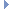OpenMM
VariableLangevinIntegrator Class Reference

This is an error contolled, variable time step Integrator that simulates a System using Langevin dynamics. More...Inheritance diagram for VariableLangevinIntegrator:

List of all members.

## Public Member Functions

def getTemperature
getTemperature(self) -> double
def setTemperature
Set the temperature of the heat bath (in Kelvin).
def getFriction
getFriction(self) -> double
def setFriction
Set the friction coefficient which determines how strongly the system is coupled to the heat bath (in inverse ps).
def getErrorTolerance
getErrorTolerance(self) -> double
def setErrorTolerance
Set the error tolerance.
def getRandomNumberSeed
getRandomNumberSeed(self) -> int
def setRandomNumberSeed
Set the random number seed.
def step
Advance a simulation through time by taking a series of time steps.
def stepTo
Advance a simulation through time by taking a series of steps until a specified time is reached.
def __init__
__init__(self, temperature, frictionCoeff, errorTol) -> VariableLangevinIntegrator __init__(self, other) -> VariableLangevinIntegrator

this

## Detailed Description

This is an error contolled, variable time step Integrator that simulates a System using Langevin dynamics.

It compares the result of the Langevin integrator to that of an explicit Euler integrator, takes the difference between the two as a measure of the integration error in each time step, and continuously adjusts the step size to keep the error below a specified tolerance. This both improves the stability of the integrator and allows it to take larger steps on average, while still maintaining comparable accuracy to a fixed step size integrator.

It is best not to think of the error tolerance as having any absolute meaning. It is just an adjustable parameter that affects the step size and integration accuracy. You should try different values to find the largest one that produces a trajectory sufficiently accurate for your purposes. 0.001 is often a good starting point.

## Constructor & Destructor Documentation

 def __init__ ( self, args )

__init__(self, temperature, frictionCoeff, errorTol) -> VariableLangevinIntegrator __init__(self, other) -> VariableLangevinIntegrator

Create a VariableLangevinIntegrator.

Parameters:
 temperature (double) the temperature of the heat bath (in Kelvin) frictionCoeff (double) the friction coefficient which couples the system to the heat bath (in inverse picoseconds) errorTol (double) the error tolerance

## Member Function Documentation

 def getErrorTolerance ( self )

getErrorTolerance(self) -> double

Get the error tolerance.

 def getFriction ( self )

getFriction(self) -> double

Get the friction coefficient which determines how strongly the system is coupled to the heat bath (in inverse ps).

Returns:
(double) the friction coefficient, measured in 1/ps
 def getRandomNumberSeed ( self )

getRandomNumberSeed(self) -> int

Get the random number seed. See setRandomNumberSeed() for details.

 def getTemperature ( self )

getTemperature(self) -> double

Get the temperature of the heat bath (in Kelvin).

Returns:
(double) the temperature of the heat bath, measured in Kelvin
 def setErrorTolerance ( self, tol )

Set the error tolerance.

 def setFriction ( self, coeff )

Set the friction coefficient which determines how strongly the system is coupled to the heat bath (in inverse ps).

Parameters:
 coeff (double) the friction coefficient, measured in 1/ps
 def setRandomNumberSeed ( self, seed )

Set the random number seed.

The precise meaning of this parameter is undefined, and is left up to each Platform to interpret in an appropriate way. It is guaranteed that if two simulations are run with different random number seeds, the sequence of random forces will be different. On the other hand, no guarantees are made about the behavior of simulations that use the same seed. In particular, Platforms are permitted to use non-deterministic algorithms which produce different results on successive runs, even if those runs were initialized identically.

If seed is set to 0 (which is the default value assigned), a unique seed is chosen when a Context is created from this Force. This is done to ensure that each Context receives unique random seeds without you needing to set them explicitly.

 def setTemperature ( self, temp )

Set the temperature of the heat bath (in Kelvin).

Parameters:
 temp (double) the temperature of the heat bath, measured in Kelvin
 def step ( self, steps )

Advance a simulation through time by taking a series of time steps.

Parameters:
 steps (int) the number of time steps to take

Reimplemented from Integrator.

 def stepTo ( self, time )

Advance a simulation through time by taking a series of steps until a specified time is reached.

When this method returns, the simulation time will exactly equal the time which was specified. If you call this method and specify a time that is earlier than the current time, it will return without doing anything.

Parameters:
 time (double) the time to which the simulation should be advanced

## Member Data Documentation

Reimplemented from Integrator.

The documentation for this class was generated from the following file:
• openmm.py